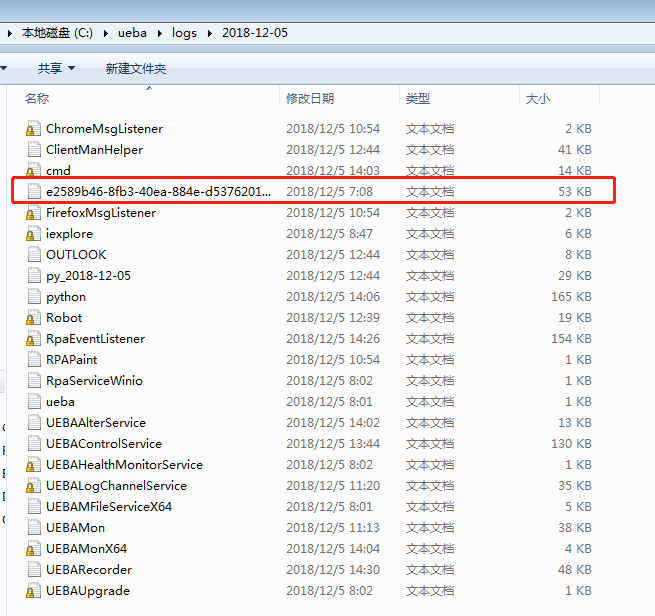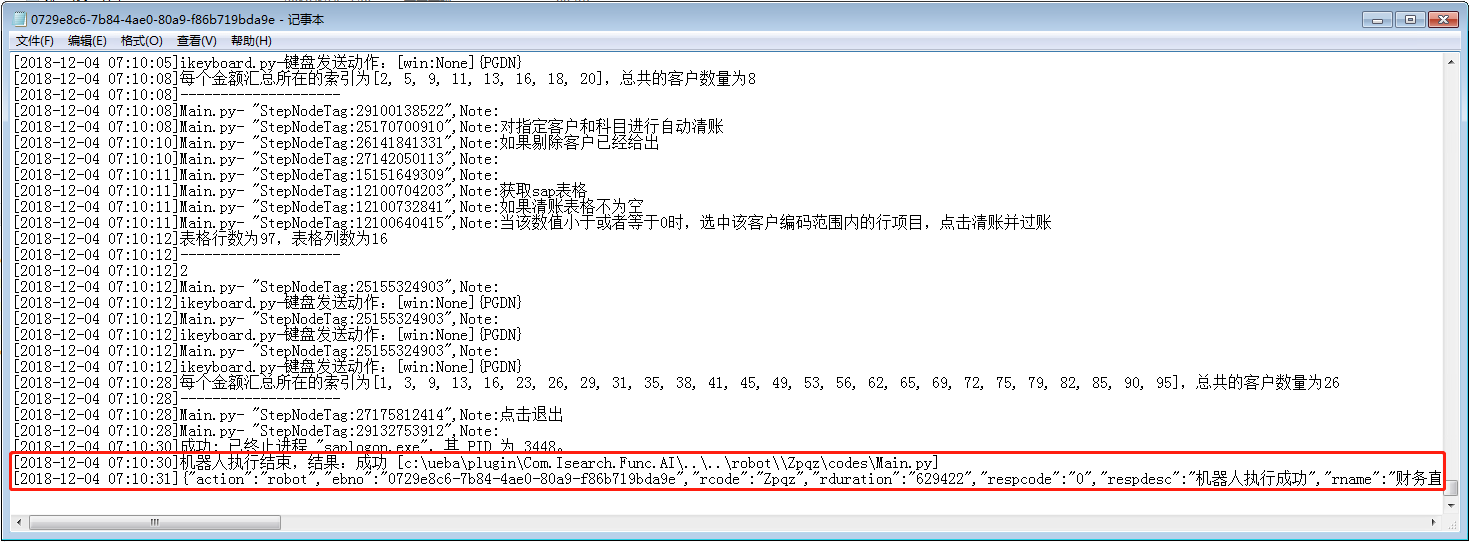# 监测云服务器机器人自动运行情况

## 问题## 思路

``````import time

path = 'C:/ueba/logs/' + str(time.localtime().tm_year) + '-' + '{:02d}'.format(time.localtime().tm_mon) + '-' + '{:02d}'.format(time.localtime().tm_mday)  # 月份和天数需要补齐两位
``````

``````import os

for i in os.listdir(path):
if len(i) == 40 and time.strftime('%H:%M:%S', time.localtime(os.stat(path + '/' + i).st_ctime)) == '07:00:00':  # 名字长度为40且创建时间为七点
path2 = path + '/' + i
``````

``````if len(path2) > 60:  # 防止文件夹中没有日志文件
try:
with open(path2, 'rb') as f:
f.seek(-1000, 2)  # 指针移动至距离末尾1000个字节的位置
lines = f.read().decode('ansi').strip().split('\n')[-1]  # txt文本默认存储格式为ANSI，需要按次格式解码，否则会报错
except:
pass
``````

``````import os
import time
import ubpa.ioutlook as ioutlook
import ubpa.ibox as ibox

path = 'C:/ueba/logs/' + str(time.localtime().tm_year) + '-' + '{:02d}'.format(time.localtime().tm_mon) + '-' + '{:02d}'.format(time.localtime().tm_mday)

for i in os.listdir(path):
if len(i) == 40 and time.strftime('%H:%M:%S', time.localtime(os.stat(path + '/' + i).st_ctime)) == '07:00:00':  # 名字长度为40且创建时间为七点
path2 = path + '/' + i

print('文件路径：', path2)
lines = ''
if len(path2) > 60:
try:
with open(path2, 'rb') as f:
f.seek(-1000, 2)
lines = f.read().decode('ansi').strip().split('\n')[-1]
except:
pass

if lines:
if '失败' in lines:
ioutlook.send_outlook(receiver='gxvdirpa4.ssc',subject='机器人执行异常',body='错误信息为：\n\n' + lines)
ibox.msg_box(msg='邮件发送成功')
elif '成功' in lines:
ibox.msg_box(msg='机器人执行正常')
``````